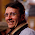## Sunday, October 11, 2009

### Puzzle game with dice

On average how many times do you need to roll dice before all the six faces appear?

#### 1 comment:

1.There are many solutions to this one. I like the following:

1. What is the probability of seeing a number ? 1/6
2. On n trials, what is the probability of seeing the k-th number, provided that you have seen (k-1)th before ? there are n-(k-1) numbers to see and so the probability is p=(n-k+1)/6
3. What is the average number of trial before a success? This is a classical question: \sum_i(i p (1-p)^(i-1)). It's a success after i-1 insuccess and that series converge to 1/p
4. Therefore we have, that for the k tentative the expectation is 6 / (n-k+1)
5. Since the expectation is linear
k=1 => 6/6
k=2 => 5/6
k=3 => 4/6
k=4 => 3/6
k=5 => 2/6
k=6 => 1/6
This last passage is very intuitive and can be given directly. The result comes from the sum of all the above fractions since expectation is linear.